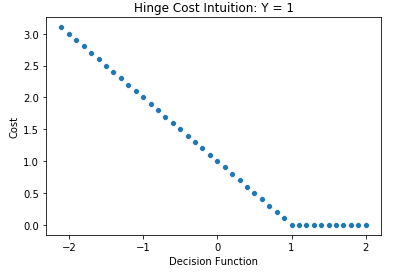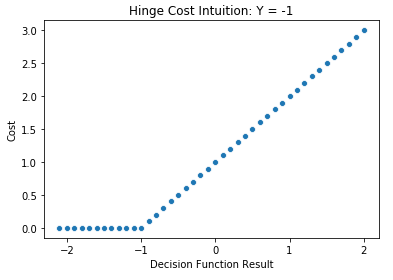# Describe the hinge loss function used in SVM

The hinge loss function uses the distance between observations and the hyperplane that separates classes in the SVM algorithm in order to quantify the magnitude of error. The formulation of the cost for a single observation is given by:

The properties of the loss function can be summarized as follows:

• Observations that are classified correctly and are at a distance beyond the margin incur no amount of error
• Observations located on the boundary of the margin incur a cost of magnitude 1, regardless of whether they are classified correctly
• Observations that reside on the correct side of the decision boundary but within the margin of error incur a cost of magnitude between 0 and 1, where those closer to the boundary receive a higher cost
• Observations located on the incorrect side of the decision boundary receive a cost greater than 1, increasing linearly with distance from the hyperplane

These properties can be seen in the simulation below. The first case shows the cost using HInge loss for an observation that belongs to the +1 class. The cost is zero when the decision function outputs a value greater than or equal to 1; the cost is 1 when it outputs zero (meaning it lies on the margin); and greater than 1 if the decision function outputs a negative value.Analogously, when the actual class is -1, the loss is 0 if the decision function produces values less than or equal to -1; equal to 1 if it outputs 0; and greater than 1 and linearly increasing for values above 0.Hinge loss is only applicable in soft margin classification, as in the case of a hard margin, there is no error, since the boundary is not allowed to have misclassifications.

Find out all the ways
that you can